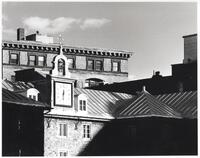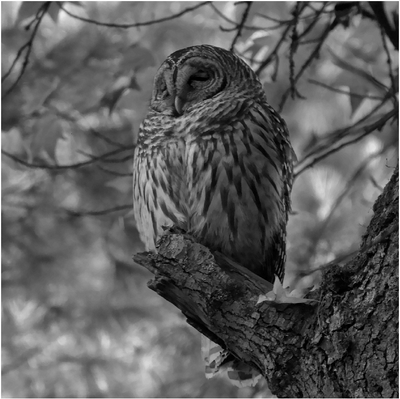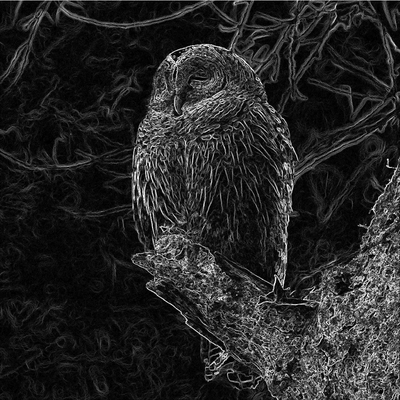UNB/ CS/ David Bremner/ teaching/ cs2613/ labs/ Lab 22

# Before the lab

• Complete the `rowfun` exercise at the end of Lab 21

Time
25 minutes
Activity
Demo/Discussion

By this point we're hopefully all pretty familiar with Octave code that looks like

```A=[1,2,3;
4,5,6];
B=A.*2
```

We're also familiar with

```C=A .* [2,2,2; 2,2,2]
```

It turns out these are actually the same operation, since Octave converts the first into the second via broadcasting.

Quoting from the Octave docs, for element-wise binary operators and functions

The rule is that corresponding array dimensions must either be equal, or one of them must be 1.

In the case where one if the dimensions is `1`, the smaller matrix is tiled to match the dimensions of the larger matrix.

Here's another example you can try.

```x = [1 2 3;
4 5 6;
7 8 9];

y = [10 20 30];

x + y
```

## Reshaping arrays

One potentially surprising aspect of Octave arrays is that the number of dimensions is independent from the number of elements. We can add as many dimensions as we like, as long as the only possible index in those dimensions is `1`. This can be particularly useful when trying to broadcast with higher dimensional arrays.

```     ones(3,3,3) .* reshape([1,2,3],[1,1,3])
ones(3,3,3) .* reshape([1,2,3],[1,3,1])
```

# Scaling layers of arrays

Time
25 minutes
Activity
Individual

Complete the following function. You may want to copy the definitions of `A` and `B` into the REPL to understand the use of `cat`.

```## usage: scale_layers(array, weights)
##
## multiply each layer of a 3D array by the corresponding weight
function out = scale_layers(array, weights)
out =
endfunction

%!test
%! onez = ones(3,3);
%! A=cat(3,onez, 2*onez, 3*onez);
%! B=cat(3,onez, 6*onez, 15*onez);
%! assert(scale_layers(A,[1;3;5]),B)
```

## Scaling a colour channelSave the image above left as `~/fcshome/teaching/cs2613/labs/L22/paris.jpg` (make sure you get the full resolution image, and not the thumbnail).

Run the following demo code; you can change the weight vector for different colourization.

```paris=imread("paris.jpg");
sepia=scale_layers(paris,[0.9,0.62,0.34]);
imshow(sepia);
```

You should get something like the following# Converting to monochrome

Time
25 minutes
Activity
Individual

Either for creative reasons, or as part of a more complex image processing task, it is common to convert images to monochrome.

Save the following image as `~/fcshome/teaching/cs2613/labs/L22/owl.jpg` (make sure you get the full resolution image, and not the thumbnail).Complete the following function using `scale_layers`.

```function out = monochrome(in, weights=[0.21,0.72,0.07])
out =
endfunction
```

Run the `monochrome` function on `owl.jpg`, you should get something like the following.Try a few different values of weight vectors, see if you can generalize some rules from your experiments.

Time
25 minutes
Activity
Individual

One useful operation on images is to detect how they are changing locally. Roughly speaking the gradient can be thought about as specifying both how much the intensity is changing, and what direction that change is happening in. Octave returns that information as an `(x,y)` vector in the usual parallel array way.

```A = [0,0,0,0,0,1;
0,1,1,1,0,0;
0,1,1,1,0,1;
0,1,1,1,1,0;
0,1,1,1,1,0;
0,0,0,0,0,0];

imshow(A);
figure;
imshow(Dx);
figure;
imshow(Dy);
```

Complete the follow function, by combining the `Dx` and `Dy` arrays into a single array such that `normimg(i,j) = norm([Dx(i,j),Dy(i,j)]). Don't use a loop. The demo should produce an image somewhat like the one below.

```function normimg = normgrad(img)